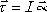tau = I TIMES alpha

The net external torque acting on an object is equal to the moment of inertia of that object times the angular acceleration of that object.

This is a big one. This is Newton's 2nd Law for rotational motion. In copying it from the formula sheet one should write it aswhich states that, for an object under the influence of torques, the sum of all the external torques about point P (where point P is identified, on a diagram, by the person solving the problem, as the axis of rotation of the object ), with counter-clockwise torques being considered positive and thus clockwise torques being considered negative, is equal to the moment of inertia of the object, times the counter-clockwise angular acceleration of the object with respect to point P.

Pitfall Avoidance Note: The left hand side of this equation is the sum of torques, not forces. When you replace the sum of torques with all the torques added together, make sure each torque is really a torque (force times moment arm with units of newtons-times-meters, not newtons), not a force.